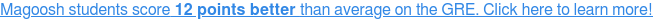offers hundreds of practice questions and video explanations. Go there now.

# Integer Properties: Practice Question of the Week #2 Answer!

Integer properties:

Ifis an non-negative integer andis a divisor ofthen=

A.B.C.D.E.The answer is B.Let us know if you need any help understanding the explanations!

See you next Tuesday for another Practice Question of the Week, happy studying!### 4 Responses to Integer Properties: Practice Question of the Week #2 Answer!

1.Jordan August 14, 2011 at 1:29 am #

Hi!
Sorry i don’t quite understand the solution to this problem. Isn’t a divisor simply a number that you divide something by. Like 20/2, 2 is the divisor. So how can 15 not be a divisor because it is a factor of 759,325. 759,325/15=66667. Not too sure what math trick that 7+5+9+3+2+5 is either. Hope you can clear some of this up.

Thanks for all the help,
-Jordan

•Margarette August 14, 2011 at 8:04 pm #

Hi, Jordan!

1. You’re right, a divisor is basically a factor of a number, and 2 is indeed a factor of 20. However, the crucial part of the definition of a divisor is that if a given number is divided by a true divisor, the answer should be a whole number (basically, no remainder left when you divide). For example, 20/2=10, with no remainder, and 10 is a whole number, so 2 is a divisor of 20. But since 759325/15=50621.66667, that means there was a remainder since 50621.666667 is not a whole number, which disqualified 15 from being a divisor.

2. the 7+5+9+3+2+5 rule is just a shortcut to quickly figure out whether a large number is divisible by 3. Chris explains the trick here: https://magoosh.com/gre/2011/gre-math-divisibility-rules-%E2%80%93-helpful-tips/. Essentially, you add up the digits in the large number: 759,325 becomes 7+5+9+3+2+5, which equals 31. Then, you check to see whether that number is divisible by 3, and since 31 definitely can’t be divided by 3, that means the original number (759,325) is also not divisible by 3.

Hope that helps– feel free to let us know if you have any other questions!

2.Karthik Chockkalingam June 9, 2011 at 7:11 am #

How the winner is selected? Is it done at random? What’s the criteria?

•margarette June 9, 2011 at 9:34 am #

Yes, we pick a random winner from the pool of readers who submitted correct answers/explanations. Although this week is probably the last week we’ll be having the “Practice Question of the Week” contest, we’ll be having other giveaways in the future, so be sure to keep checking in with the blog!

Magoosh blog comment policy: To create the best experience for our readers, we will only approve comments that are relevant to the article, general enough to be helpful to other students, concise, and well-written! 😄 Due to the high volume of comments across all of our blogs, we cannot promise that all comments will receive responses from our instructors.

We highly encourage students to help each other out and respond to other students' comments if you can!

If you are a Premium Magoosh student and would like more personalized service from our instructors, you can use the Help tab on the Magoosh dashboard. Thanks!